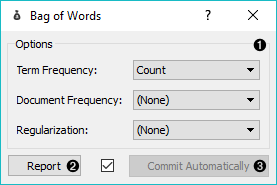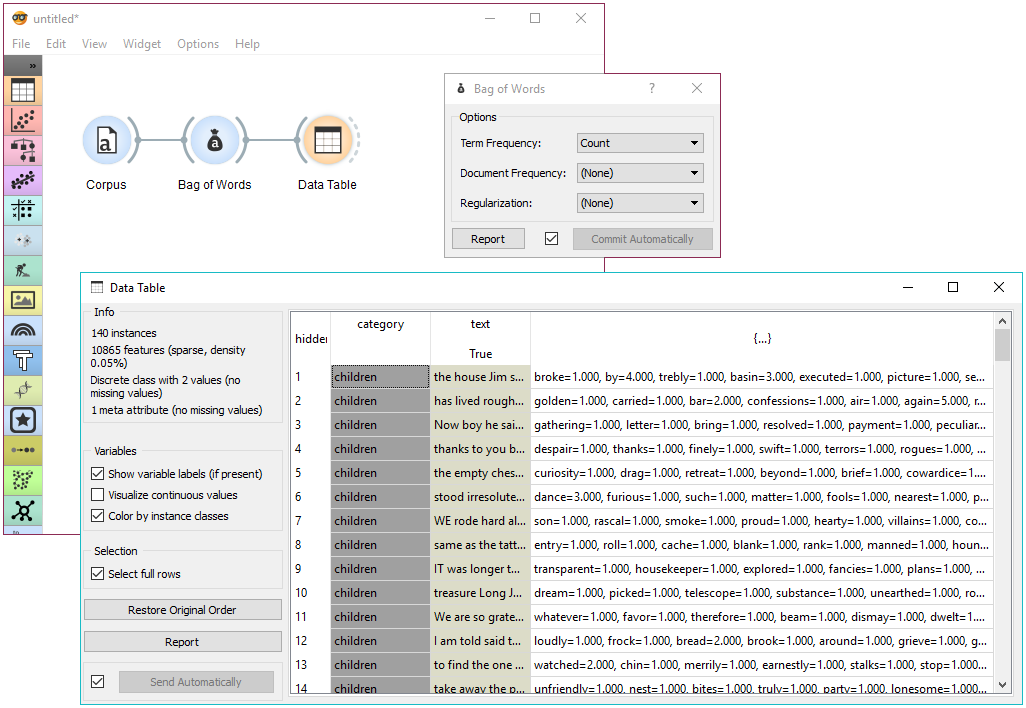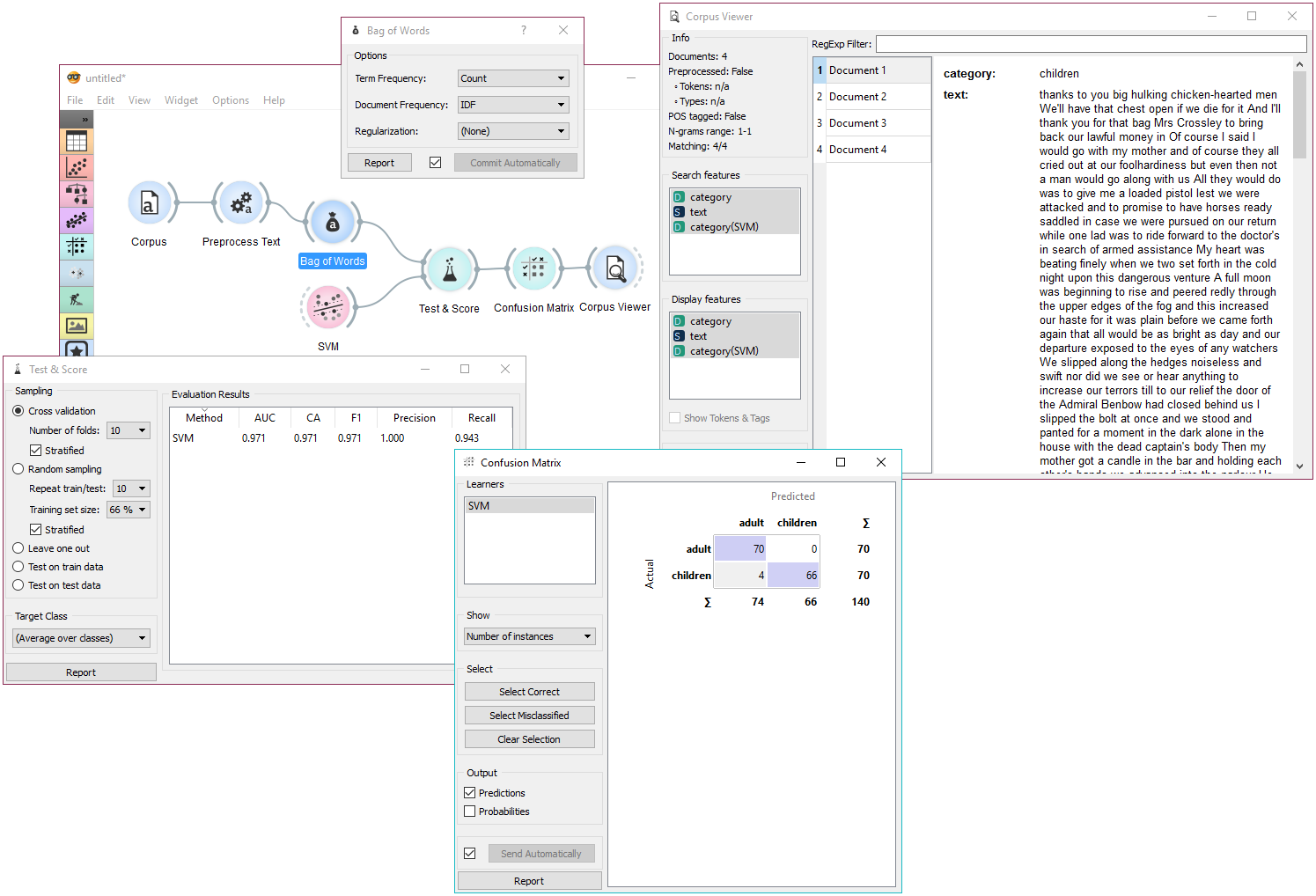# Bag of Words

Generates a bag of words from the input corpus.

Inputs

• Corpus: A collection of documents.

Outputs

• Corpus: Corpus with bag of words features appended.

Bag of Words model creates a corpus with word counts for each data instance (document). The count can be either absolute, binary (contains or does not contain) or sublinear (logarithm of the term frequency). Bag of words model is required in combination with Word Enrichment and could be used for predictive modelling.1. Parameters for bag of words model:
• Term Frequency:
• Count: number of occurrences of a word in a document
• Binary: word appears or does not appear in the document
• Sublinear: logarithm of term frequency (count)
• Document Frequency:
• Regulariation:
• (None)
• L1 (Sum of elements): normalizes vector length to sum of elements
• L2 (Euclidean): normalizes vector length to sum of squares
2. Produce a report.
3. If Commit Automatically is on, changes are communicated automatically. Alternatively press Commit.

## Example

In the first example we will simply check how the bag of words model looks like. Load book-excerpts.tab with Corpus widget and connect it to Bag of Words. Here we kept the defaults - a simple count of term frequencies. Check what the Bag of Words outputs with Data Table. The final column in white represents term frequencies for each document.In the second example we will try to predict document category. We are still using the book-excerpts.tab data set, which we sent through Preprocess Text with default parameters. Then we connected Preprocess Text to Bag of Words to obtain term frequencies by which we will compute the model.Connect Bag of Words to Test & Score for predictive modelling. Connect SVM or any other classifier to Test & Score as well (both on the left side). Test & Score will now compute performance scores for each learner on the input. Here we got quite impressive results with SVM. Now we can check, where the model made a mistake.

Add Confusion Matrix to Test & Score. Confusion matrix displays correctly and incorrectly classified documents. Select Misclassified will output misclassified documents, which we can further inspect with Corpus Viewer.